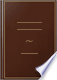Søk Bilder Maps Play YouTube Nyheter Gmail Disk Mer »
Logg på
 Bøker Bok 1–10 av 59 på Divide a straight line into two parts such that the rectangle contained by the whole...Divide a straight line into two parts such that the rectangle contained by the whole line and one of the parts shall be equal to the square on the other part.An Elementary Treatise on the Geometrical and Algebraical Investigation of ... - Side 85
av Daniel Cresswell - 1817 - 436 sider
Uten tilgangsbegrensning - Om denne boken## A Supplement to the Elements of Euclid

Daniel Cresswell - 1819 - 410 sider
...(Constr.) AB is to AD in the given ratio ; . -. PQ is to PR in the given ratio. PROP. XLII. 51. PROBLEM. To divide a given straight line into two parts, such,...have a given ratio to the square of the other part. Let AB be the given straight line : It is reA r, quired to divide it into two parts, such that the...
Uten tilgangsbegrensning - Om denne boken## A Supplement to the Elements of Euclid

Daniel Cresswell - 1825 - 582 sider
...(constr.) AB is to AD in the given ratio ; ... PQ is to PR in the given ratio. PROP. XLII. 51. PROBLEM. To divide a given straight line into two parts, such, that the rectangle contained by the whole tine and out; of Us parts, shall have a given ratio to the square of the other part *. Let Jli be the...
Uten tilgangsbegrensning - Om denne boken## Elements of Geometry: With Notes

John Radford Young - 1827 - 208 sider
...is included, without violating one of the most obvious rules of logic. PROPOSITION XXXII. PROBLEM. To divide a given straight line into two parts, such that the greater part may be a mean proportional between the whole line and the other part. Let AB be the proposed...
Uten tilgangsbegrensning - Om denne boken## The First Six and the Eleventh and Twelfth Books of Euclid's Elements: With ...

Euclid - 1837 - 390 sider
...the lines forming a given angle, to draw a straight line cutting off the least possible triangle. 40. To divide a given straight line into two parts, such that the square of one of them may be double of the square of the other, or may be in any given ratio to it....
Uten tilgangsbegrensning - Om denne boken## The first six and the eleventh and twelfth books of Euclid's Elements; with ...

James Thomson - 1845 - 358 sider
...(I. 47) equal to the squares of GA, AM, the sides of the hexagon and decagon. PROP. XXII. PROB. — To divide a given straight line into two parts, such that the square of one of them may be equal to the rectangle contained by the other, and a given straight line....
Uten tilgangsbegrensning - Om denne boken## durham university calendar, 1851

77 - 1851
...line is equal to the square of the parts together with twice the rectangle contained by the parts. 5. To divide a given straight line into two parts, such that the rectangle contained by the whole and one of the parts shall be equal to the square of the other part. 6. If two circles cut one another...
Uten tilgangsbegrensning - Om denne boken## Arithmetic, algebra, differential and integral calculus, by W. Rutherford ...

...both are answers to the question in its literal meaning. 2. To divide a line of 20 inches in length into two parts such that the rectangle contained by the whole line and one of the parts shall be equal to the square of the other part. Let x = the greater part ; then 20 — x...
Uten tilgangsbegrensning - Om denne boken## The Elements of geometry; or, The first six books, with the eleventh and ...

Euclides - 1855
...they meet the circumference, are together equal to the square of the diameter. PROP. I)- PROBLEM — To divide a given straight line into two parts, such that the square of one of them may be equal to the rectangle contained by the other and a given straight line....
Uten tilgangsbegrensning - Om denne boken## Gradations in Euclid : books i. and ii., with an explanatory preface [&c ...

Euclides - 1858
...figure ABED is the square required. (46. I.) PBOB. 15. To divide a given line, AB, into two parts, so that the rectangle contained by the whole line and one of its parte, AB-BH, Khali be equal to the square of the other part, AH. On AB, by 46. 1. , describe the square...
Uten tilgangsbegrensning - Om denne boken## The examination papers as set for the preliminary literary examination of ...

...bisected, shall be equal to the square of the straight line made up of the half and the part produced. 8. Divide a given straight line into two parts, such...the rectangle contained by the whole line and one of the parts may be equal to the square of the other part. PRELIMINARY GENERAL EXAMINATION. Christmas,...
Uten tilgangsbegrensning - Om denne boken